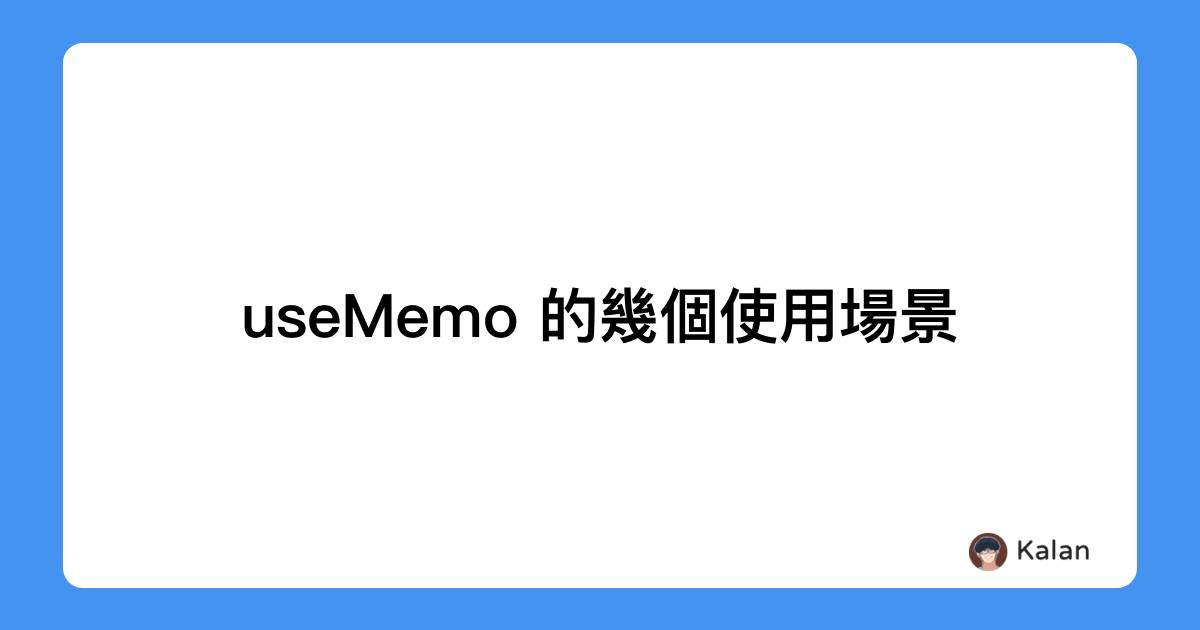# useMemo 的幾個使用場景## useMemo 的幾個使用場景

• 時間：將秒轉換為 xx:xx 分秒的形式顯示
• 如果勾選篩選器選項，則資料會先經過處理再顯示

## 當作 computed prop 使用

jsxconst FormatTime = (ts) => {  const formattedTime = ${Math.floor(ts/60)}:${ts%60}  return <time>{formattedTime}</time>}

jsxconst FormatTime = (ts) => {  const formattedTime = useMemo(() => {    return ${Math.floor(ts/60)}:${ts%60}  }, [ts])    return <time>{formattedTime}</time>}

jsxconst Component = (ts) => {	const computed = calculateMyProp(ts)  return <div>...</div>}

## 運算依賴於數個狀態

jsxconst MyComponent = ({ data }) => {  const [filtered, setFiltered] = useState(false)    return <div>    <button onClick={() => setFiltered(s => !s)}>toggle filtered</button>    {data.filter(d => filtered ? d.favorite : true).map(...)}  </div>}

diffconst MyComponent = ({ data }) => {  const [filtered, setFiltered] = useState(false)+ const filteredData = data.filter(d => filtered ? d.favorite : true)  return <div>    <button onClick={() => setFiltered(s => !s)}>toggle filtered</button>+		{filterData.map(...)}  </div>}

jsxconst MyComponent = ({ data }) => {  const [filtered, setFiltered] = useState(false)  const [sorted, setSorted] = useState(false)  const filteredData = useMemo(() => {    if (filtered) {	    return data.filter(d => d.favorite)          }    return data  }, [data.length, filtered])  return <div>    <button onClick={() => setFiltered(s => !s)}>toggle filtered</button>  	{filterData.map(...)}  </div>}

jsxconst MyComponent = ({ data }) => {  const [filtered, setFiltered] = useState(false)  const [sorted, setSorted] = useState(false)  const filteredData = useMemo(() => {    const origin = [...data]    if (filtered) {	    return origin.filter(d => d.favorite)          }    if (sorted) {      return data.sort()    }    return origin  }, [data.length, filtered, sorted]) // 這邊假設 data 的變化只發生在長度有變化時  return <div>    <button onClick={() => setFiltered(s => !s)}>toggle filtered</button>  	{filterData.map(...)}  </div>}

## derived state

jsxconst MyComponent = ({ data }) => {  const [query, setQuery] = useState(value)  const [filteredData, setFiltered] = useState(data.filter(d => query ? d.includes(query) : true))  return <>    <input onChange={e => setQuery(e.target.value)} value={query} />  	{filteredData.map(...)}  </>}

• 每次 data 有變化時，因為還要再更新一次狀態，多了一次不必要的更新
• 輸入值同時仰賴於 prop 與 state 造成 single source of truth 無法維持。

diff/* 不推薦此寫法 */const MyComponent = ({ data }) => {  const [query, setQuery] = useState(value)  const [filteredData, setFiltered] = useState(data.filter(d => query ? d.includes(query) : true))+ useEffect(() => { data.filter(d => d.includes(query)) }, [data, query])  return <>    <input onChange={e => setQuery(e.target.value)} value={query} />  	{filteredData.map(...)}  </>}

jsxconst MyComponent = ({ data }) => {  const [query, setQuery] = useState(value)  const filteredData = useMemo(() => {    if (query) {      return data.filter(d => d.includes(query))    }        return data  }, [data, query])	  return <>    <input onChange={e => setQuery(e.target.value)} value={query} />  	{filteredData.map(...)}  </>}

## 總結

• computed props 可以用 useMemo 幫助其他開發者減低閱讀程式碼的認知負擔

• 在運算量較大的情況下也可以節省每次渲染都要重新運算的成本
• 使用 useState(props) 時需要特別小心
• derived state 在大部分情況下可以搭配 useMemo 解決